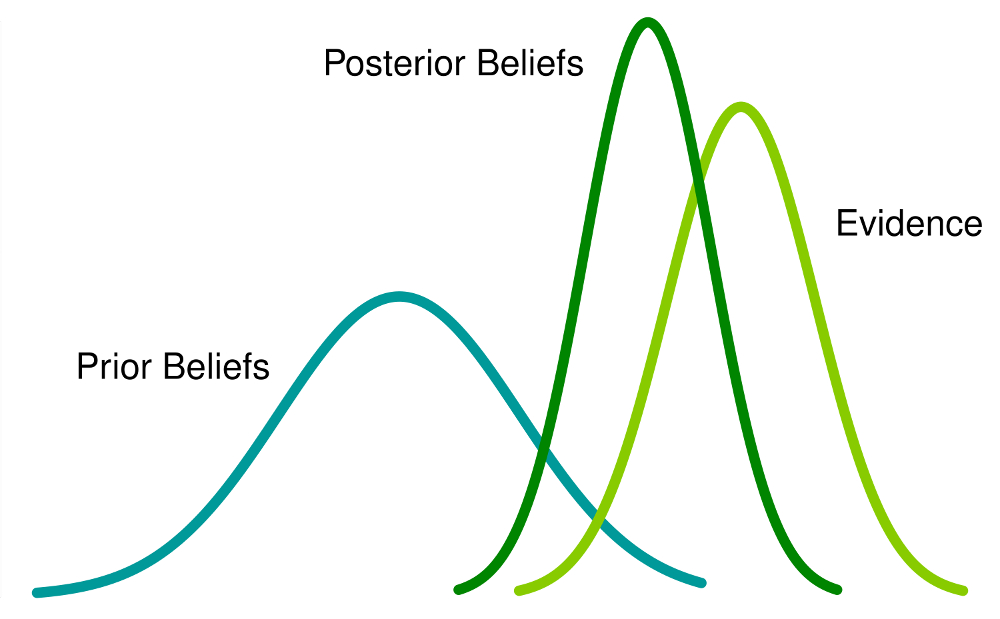## Bayesian Statistics explained to Beginners in Simple English

Bayesian Statistics continues to remain incomprehensible in the ignited minds of many analysts. Being amazed by the incredible power of machine learning, a lot of us have become unfaithful to statistics. Our focus has narrowed down to exploring machine learning. Isn’t it true?

We fail to understand that machine learning is only one way to solve real world problems. In several situations, it does not help us solve business problems, even though there is data involved in these problems. To say the least, knowledge of statistics will allow you to work on complex analytical problems, irrespective of the size of data.

In 1770s, Thomas Bayes introduced ‘Bayes Theorem’. Even after centuries later, the importance of ‘Bayesian Statistics’ hasn’t faded away. In fact, today this topic is being taught in great depths in some of the world’s leading universities.

With this idea, I’ve created this beginner’s guide on Bayesian Statistics. I’ve tried to explain the concepts in a simplistic manner with examples. Prior knowledge of basic probability & statistics is desirable. By the end of this article, you will have a concrete understanding of Bayesian Statistics and its associated concepts.1. Frequentist Statistics
2. The Inherent Flaws in Frequentist Statistics
3. Bayesian Statistics
• Conditional Probability
• Bayes Theorem
4. Bayesian Inference
• Bernoulli likelihood function
• Prior Belief Distribution
• Posterior belief Distribution
5. Test for Significance – Frequentist vs Bayesian
• p-value
• Confidence Intervals
• Bayes Factor
• High Density Interval (HDI)

More here